Do you like the article?
Share it with others -
post a link to it!
Use new possibilities of MetaTrader 5

# Graphical Interfaces X: The Standard Chart Control (build 4)

11 November 2016, 08:45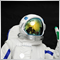0
24 356

### Introduction

The first article Graphical Interfaces I: Preparation of the Library Structure (Chapter 1) explains in detail what this library is for. You will find a list of articles with links at the end of each chapter. There, you can also download a complete version of the library at the current stage of development. The files must be placed in the same directories as they are located in the archive.

Let us consider another control, which could not be left out of the library under development. When starting the trading terminal, price charts are opened to the user. It would be convenient to have a tool that would allow to manage the charts with more ease. Previous article named MQL5 Cookbook: Monitoring Multiple Time Frames in a Single Window had demonstrated one of the possible variants of such a tool. This time, we will write a class for creating a control, that will be simple and easy to use in graphical interfaces of custom MQL applications. Unlike the previous version provided at the link above, this implementation will allow to scroll the contents of the subcharts horizontally, as in the conventional main chart window.

In addition, we will continue to optimize the library code in order to reduce the CPU load. More details are provided further in the article.

### Developing a Class for Creating the Standard Chart Control

Before starting the development of the CStandardChart class for creating the Standard Chart control, the CSubChart base class with additional properties (see code listing below) should be added to the Object.mqh file. This had been done earlier for all types of graphical objects, which are used when creating library controls. The base class for the CSubChart class is a class from the standard library — CChartObjectSubChart

```//+------------------------------------------------------------------+
//|                                                      Objects.mqh |
//|                        Copyright 2015, MetaQuotes Software Corp. |
//|                                              http://www.mql5.com |
//+------------------------------------------------------------------+
...
//--- List of classes in file for quick navigation (Alt+G)
...
class CSubChart;
//+------------------------------------------------------------------+
//| Class with additional properties for the Subchart object         |
//+------------------------------------------------------------------+
class CSubChart : public CChartObjectSubChart
{
protected:
int               m_x;
int               m_y;
int               m_x2;
int               m_y2;
int               m_x_gap;
int               m_y_gap;
int               m_x_size;
int               m_y_size;
bool              m_mouse_focus;
//---
public:
CSubChart(void);
~CSubChart(void);
//--- Coordinates
int               X(void)                      { return(m_x);           }
void              X(const int x)               { m_x=x;                 }
int               Y(void)                      { return(m_y);           }
void              Y(const int y)               { m_y=y;                 }
int               X2(void)                     { return(m_x+m_x_size);  }
int               Y2(void)                     { return(m_y+m_y_size);  }
//--- Margins from the edge point (xy)
int               XGap(void)                   { return(m_x_gap);       }
void              XGap(const int x_gap)        { m_x_gap=x_gap;         }
int               YGap(void)                   { return(m_y_gap);       }
void              YGap(const int y_gap)        { m_y_gap=y_gap;         }
//--- Size
int               XSize(void)                  { return(m_x_size);      }
void              XSize(const int x_size)      { m_x_size=x_size;       }
int               YSize(void)                  { return(m_y_size);      }
void              YSize(const int y_size)      { m_y_size=y_size;       }
//--- Focus
bool              MouseFocus(void)             { return(m_mouse_focus); }
void              MouseFocus(const bool focus) { m_mouse_focus=focus;   }
};
//+------------------------------------------------------------------+
//| Constructor                                                      |
//+------------------------------------------------------------------+
CSubChart::CSubChart(void) : m_x(0),
m_y(0),
m_x2(0),
m_y2(0),
m_x_gap(0),
m_y_gap(0),
m_x_size(0),
m_y_size(0),
m_mouse_focus(false)
{
}
//+------------------------------------------------------------------+
//| Destructor                                                       |
//+------------------------------------------------------------------+
CSubChart::~CSubChart(void)
{
}
```

The CChartObjectSubChart class contains the method for creating a subchart, as well as the methods for modifying the most frequently used chart properties. The list includes methods for setting and getting such properties as:

• coordinates and dimensions
• symbol, timeframe and scale
• display of price and time scales.
```//+------------------------------------------------------------------+
//|                                          ChartObjectSubChart.mqh |
//|                   Copyright 2009-2013, MetaQuotes Software Corp. |
//|                                              http://www.mql5.com |
//+------------------------------------------------------------------+
#include "ChartObject.mqh"
//+------------------------------------------------------------------+
//| Class CChartObjectSubChart.                                      |
//| Purpose: Class of the "SubChart" object of chart.                |
//|          Derives from class CChartObject.                        |
//+------------------------------------------------------------------+
class CChartObjectSubChart : public CChartObject
{
public:
CChartObjectSubChart(void);
~CChartObjectSubChart(void);
//--- methods of access to properties of the object
int               X_Distance(void) const;
bool              X_Distance(const int X) const;
int               Y_Distance(void) const;
bool              Y_Distance(const int Y) const;
ENUM_BASE_CORNER  Corner(void) const;
bool              Corner(const ENUM_BASE_CORNER corner) const;
int               X_Size(void) const;
bool              X_Size(const int size) const;
int               Y_Size(void) const;
bool              Y_Size(const int size) const;
string            Symbol(void) const;
bool              Symbol(const string symbol) const;
int               Period(void) const;
bool              Period(const int period) const;
int               Scale(void) const;
bool              Scale(const int scale) const;
bool              DateScale(void) const;
bool              DateScale(const bool scale) const;
bool              PriceScale(void) const;
bool              PriceScale(const bool scale) const;
//--- change of time/price coordinates is blocked
bool              Time(const datetime time) const { return(false); }
bool              Price(const double price) const { return(false); }
//--- method of creating object
bool              Create(long chart_id,const string name,const int window,
const int X,const int Y,const int sizeX,const int sizeY);
//--- method of identifying the object
virtual int       Type(void) const { return(OBJ_CHART); }
//--- methods for working with files
virtual bool      Save(const int file_handle);
virtual bool      Load(const int file_handle);
};
```

Now we can create the StandardChart.mqh file with the CStandardChart class, where the standard methods for all library controls can be specified in the base content, as shown in the code below. Since the control will feature horizontal scrolling mode, it will require an icon for mouse cursor to tell the user that scrolling mode is enabled and the data in the subchart will be shifted as the mouse cursor moves horizontally. To change the icon, include the Pointer.mqh file containing the CPointer class, which has been previously considered in the article Graphical Interfaces VIII: The Tree View Control (Chapter 2). As the icon for the mouse pointer, we will use a copy of the one that is activated with the horizontal scrolling of the main chart (double-sided black arrow with a white outline). Two versions of this icon (black and blue arrows) are attached at the end of the article.

Accordingly, the enumeration of mouse pointers (ENUM_MOUSE_POINTER) was supplemented with another identifier (MP_X_SCROLL):

```//+------------------------------------------------------------------+
//| Enumeration of the pointer types                                 |
//+------------------------------------------------------------------+
enum ENUM_MOUSE_POINTER
{
MP_CUSTOM     =0,
MP_X_RESIZE   =1,
MP_Y_RESIZE   =2,
MP_XY1_RESIZE =3,
MP_XY2_RESIZE =4,
MP_X_SCROLL   =5
};
```

In addition, it is necessary to include the resources with icons for this type of cursor in the Pointer.mqh file, and the switch construction in the CPointer::SetPointerBmp() method should be expanded with another case block

```//+------------------------------------------------------------------+
//|                                                      Pointer.mqh |
//|                        Copyright 2015, MetaQuotes Software Corp. |
//|                                              http://www.mql5.com |
//+------------------------------------------------------------------+
#include "Element.mqh"
//--- Resources
...
#resource "\\Images\\EasyAndFastGUI\\Controls\\pointer_x_scroll.bmp"
#resource "\\Images\\EasyAndFastGUI\\Controls\\pointer_x_scroll_blue.bmp"
//+------------------------------------------------------------------+
//| Set the cursor images based on cursor type                       |
//+------------------------------------------------------------------+
void CPointer::SetPointerBmp(void)
{
switch(m_type)
{
case MP_X_RESIZE :
m_file_on  ="Images\\EasyAndFastGUI\\Controls\\pointer_x_rs_blue.bmp";
m_file_off ="Images\\EasyAndFastGUI\\Controls\\pointer_x_rs.bmp";
break;
case MP_Y_RESIZE :
m_file_on  ="Images\\EasyAndFastGUI\\Controls\\pointer_y_rs_blue.bmp";
m_file_off ="Images\\EasyAndFastGUI\\Controls\\pointer_y_rs.bmp";
break;
case MP_XY1_RESIZE :
m_file_on  ="Images\\EasyAndFastGUI\\Controls\\pointer_xy1_rs_blue.bmp";
m_file_off ="Images\\EasyAndFastGUI\\Controls\\pointer_xy1_rs.bmp";
break;
case MP_XY2_RESIZE :
m_file_on  ="Images\\EasyAndFastGUI\\Controls\\pointer_xy2_rs_blue.bmp";
m_file_off ="Images\\EasyAndFastGUI\\Controls\\pointer_xy2_rs.bmp";
break;
case MP_X_SCROLL :
m_file_on  ="Images\\EasyAndFastGUI\\Controls\\pointer_x_scroll_blue.bmp";
m_file_off ="Images\\EasyAndFastGUI\\Controls\\pointer_x_scroll.bmp";
break;
}
//--- If custom type (MP_CUSTOM) is specified
if(m_file_on=="" || m_file_off=="")
::Print(__FUNCTION__," > Both images must be set for the cursor!");
}
```

It should also be noted that the Moving() method can also be used in two modes, which can be set by the third argument of the method. The default value of this argument is false, which means that a control can be moved only in case the form it is attached to is also currently in movement mode.

```//+------------------------------------------------------------------+
//|                                                StandardChart.mqh |
//|                        Copyright 2015, MetaQuotes Software Corp. |
//|                                              http://www.mql5.com |
//+------------------------------------------------------------------+
#include "Element.mqh"
#include "Window.mqh"
#include "Pointer.mqh"
//+------------------------------------------------------------------+
//| Class for creating a standard chart                              |
//+------------------------------------------------------------------+
class CStandardChart : public CElement
{
private:
//--- Pointer to the form to which the element is attached
CWindow          *m_wnd;
//---
public:
//--- Stores the form pointer
void              WindowPointer(CWindow &object)          { m_wnd=::GetPointer(object); }
//---
public:
//--- Chart event handler
virtual void      OnEvent(const int id,const long &lparam,const double &dparam,const string &sparam);
//--- Moving the element
virtual void      Moving(const int x,const int y,const bool moving_mode=false);
//--- (1) Show, (2) hide, (3) reset, (4) delete
virtual void      Show(void);
virtual void      Hide(void);
virtual void      Reset(void);
virtual void      Delete(void);
//--- (1) Set, (2) reset priorities of the left mouse button press
virtual void      SetZorders(void);
virtual void      ResetZorders(void);
//---
private:
//--- Change the width at the right edge of the window
virtual void      ChangeWidthByRightWindowSide(void);
//--- Change the height at the bottom edge of the window
virtual void      ChangeHeightByBottomWindowSide(void);
};
//+------------------------------------------------------------------+
//| Constructor                                                      |
//+------------------------------------------------------------------+
CStandardChart::CStandardChart(void)
{
//--- Store the name of the element class in the base class
CElement::ClassName(CLASS_NAME);
}
//+------------------------------------------------------------------+
//| Destructor                                                       |
//+------------------------------------------------------------------+
CStandardChart::~CStandardChart(void)
{
}
```

If the value of the third argument in the Moving() method is set to true, then the control will be moved forcibly upon calling the method, regardless of whether the form is in the movement mode. In some cases, this significantly reduces the CPU load.

In order to see if the form is in movement mode, the ClampingAreaMouse() method has been added to the CWindow class. It returns the area where the left mouse button had been pressed:

```//+------------------------------------------------------------------+
//| Class for creating a form for controls                           |
//+------------------------------------------------------------------+
class CWindow : public CElement
{
public:
//--- Returns the are where the left mouse button was pressed
ENUM_MOUSE_STATE  ClampingAreaMouse(void)                           const { return(m_clamping_area_mouse);          }
};
```

The CWindow::ClampingAreaMouse() method can only be accessed via the form pointer in each control attached to the form. For everything to work as described above, a code block should be inserted to the Moving() method of each control, as shown in the listing below (see the fragment highlighted in yellow). As an example, the method from the CSimpleButton (short version) class is shown.

```//+------------------------------------------------------------------+
//| Moving controls                                                  |
//+------------------------------------------------------------------+
void CSimpleButton::Moving(const int x,const int y,const bool moving_mode=false)
{
//--- Leave, if the element is hidden
if(!CElement::IsVisible())
return;
//--- If the management is delegated to the window, identify its location
if(!moving_mode)
if(m_wnd.ClampingAreaMouse()!=PRESSED_INSIDE_HEADER)
return;
//--- If the anchored to the right
//--- If the anchored to the left
//--- If the anchored to the bottom
//--- If the anchored to the top
//--- Updating coordinates of graphical objects
m_button.X_Distance(m_button.X());
m_button.Y_Distance(m_button.Y());
}
```

Example of using the Moving() method can be seen in the listing below, which demonstrates the code of the CSimpleButton::Show() method. In this case, the control coordinates should be updated forcibly, therefore, true was passed as the third argument. Appropriate changes were made to all the library classes, which use the Moving() method.

```//+------------------------------------------------------------------+
//| Shows the button                                                 |
//+------------------------------------------------------------------+
void CSimpleButton::Show(void)
{
//--- Leave, if the element is already visible
if(CElement::IsVisible())
return;
//--- Make all objects visible
m_button.Timeframes(OBJ_ALL_PERIODS);
//--- State of visibility
CElement::IsVisible(true);
//--- Update the position of objects
Moving(m_wnd.X(),m_wnd.Y(),true);
}
```

Optimization of the developed library will be discussed later in the article, as for now, the Standard Chart control will be considered.

Let us make it possible to create an array of subcharts placed in a row. For this, it is necessary to declare dynamic arrays for objects that represent charts, as well as for certain properties, such as: (1) chart identifier, (2) symbol and (3) timeframe. Before creating the Standard Chart control, it is necessary to use the CStandardChart::AddSubChart() method, where the chart symbol and timeframe should be passed. At the beginning of this method, before adding the elements to the arrays and initializing them with the passed values, there is a check for symbol availability using the CStandardChart::CheckSymbol() method.

```class CStandardChart : public CElement
{
private:
//--- Objects for creating the element
CSubChart         m_sub_chart[];
//--- Chart properties:
long              m_sub_chart_id[];
string            m_sub_chart_symbol[];
ENUM_TIMEFRAMES   m_sub_chart_tf[];
//---
public:
//--- Adds a chart with specified properties before creation
void              AddSubChart(const string symbol,const ENUM_TIMEFRAMES tf);
//---
private:
//--- Checking the symbol
bool              CheckSymbol(const string symbol);
};
```

The CStandardChart::CheckSymbol() method first checks if the specified symbol is available in the Market Watch window. If the symbol was not found, it them attempts to find the symbol in the general list. If the symbol is found, then it must be added to the Market Watch. Otherwise, it will be impossible to create the subchart with this symbol (a subchart with the symbol from the main chart window will be created instead).

If succeeded, the CStandardChart::CheckSymbol() method returns true. If the specified symbol was not found, the method will return false, and the subchart will not be added (arrays will have the same size), a message about that will be displayed in the journal.

```//+------------------------------------------------------------------+
//| Adds a chart                                                     |
//+------------------------------------------------------------------+
void CStandardChart::AddSubChart(const string symbol,const ENUM_TIMEFRAMES tf)
{
//--- Check if the symbol is available on the server
if(!CheckSymbol(symbol))
{
::Print(__FUNCTION__," > Symbol "+symbol+" is not available on the server!");
return;
}
//--- Increase the array size by one element
int array_size=::ArraySize(m_sub_chart);
int new_size=array_size+1;
::ArrayResize(m_sub_chart,new_size);
::ArrayResize(m_sub_chart_id,new_size);
::ArrayResize(m_sub_chart_symbol,new_size);
::ArrayResize(m_sub_chart_tf,new_size);
//--- Store the value of passed parameters
m_sub_chart_symbol[array_size] =symbol;
m_sub_chart_tf[array_size]     =tf;
}
//+------------------------------------------------------------------+
//| Checking the symbol availability                                 |
//+------------------------------------------------------------------+
bool CStandardChart::CheckSymbol(const string symbol)
{
bool flag=false;
//--- Check for the symbol in the Market Watch
int symbols_total=::SymbolsTotal(true);
for(int i=0; i<symbols_total; i++)
{
//--- If the symbol is available, stop the cycle
if(::SymbolName(i,true)==symbol)
{
flag=true;
break;
}
}
//--- If the symbol is not available in the Market Watch window
if(!flag)
{
//--- ... attempt to find it in the general list
symbols_total=::SymbolsTotal(false);
for(int i=0; i<symbols_total; i++)
{
//--- If this symbol is available
if(::SymbolName(i,false)==symbol)
{
//--- ... add it to the Market Watch window and stop the cycle
::SymbolSelect(symbol,true);
flag=true;
break;
}
}
}
//--- Return the search results
return(flag);
}
```

Creating a Standard Chart control will require three methods: one main public method and two private methods, one of which refers to the icon for mouse cursor in horizontal scrolling mode. The CStandardChart::SubChartsTotal() public method has been added to the class as an auxiliary method for retrieving the number of subcharts.

```class CStandardChart : public CElement
{
private:
//--- Objects for creating the element
CSubChart         m_sub_chart[];
CPointer          m_x_scroll;
//---
public:
//--- Methods for creating a standard chart
bool              CreateStandardChart(const long chart_id,const int subwin,const int x,const int y);
//---
private:
bool              CreateSubChart(void);
bool              CreateXScrollPointer(void);
//---
public:
//--- Returns the size of the charts array
int               SubChartsTotal(void)              const { return(::ArraySize(m_sub_chart)); }
};
```

Let us consider the CStandardChart::CreateSubCharts() method for creating subcharts. Here, a check for the number of charts added to the arrays before creating the control is at the beginning of the method. If none was added, the program simply leaves the method and prints a relevant message in the journal.

If charts were added, then the width is calculated for each object. The total width of a control must be defined by user in the custom class of the developed MQL application. In case it is necessary to create multiple charts, then it is sufficient to divide the total width of the control by the number of charts in order to obtain the width for each object.

Then the objects are created in a loop. This takes into account the control positioning (anchor points to one of the form sides). This topic has been thoroughly described in the previous article, therefore it will not be discussed here.

After creating the subchart, the identifier of the created chart is obtained and stored to the array, its properties are set and stored.

```//+------------------------------------------------------------------+
//| Create charts                                                    |
//+------------------------------------------------------------------+
bool CStandardChart::CreateSubCharts(void)
{
//--- Get the number of charts
int sub_charts_total=SubChartsTotal();
//--- If there is no chart in the group, report
if(sub_charts_total<1)
{
::Print(__FUNCTION__," > This method is to be called, "
"if a group contains at least one chart! Use the CStandardChart::AddSubChart() method");
return(false);
}
//--- Calculate the coordinates and size
int x=m_x;
int x_size=(sub_charts_total>1)? m_x_size/sub_charts_total : m_x_size;
//--- Create the specified number of charts
for(int i=0; i<sub_charts_total; i++)
{
//--- Forming the object name
string name=CElement::ProgramName()+"_sub_chart_"+(string)i+"__"+(string)CElement::Id();
//--- Calculation of the X coordinate
x=(i>0)?(m_anchor_right_window_side)? x-x_size+1 :  x+x_size-1 : x;
//--- Adjust the width of the last chart
if(i+1>=sub_charts_total)
x_size=m_x_size-(x_size*(sub_charts_total-1)-(sub_charts_total-1));
//--- Set up a button
if(!m_sub_chart[i].Create(m_chart_id,name,m_subwin,x,m_y,x_size,m_y_size))
return(false);
//--- Get and store the identifier of the created chart
m_sub_chart_id[i]=m_sub_chart[i].GetInteger(OBJPROP_CHART_ID);
//--- set properties
m_sub_chart[i].Symbol(m_sub_chart_symbol[i]);
m_sub_chart[i].Period(m_sub_chart_tf[i]);
m_sub_chart[i].Z_Order(m_zorder);
m_sub_chart[i].Tooltip("\n");
//--- Store the size
m_sub_chart[i].XSize(x_size);
m_sub_chart[i].YSize(m_y_size);
//--- Margins from the edge
m_sub_chart[i].XGap((m_anchor_right_window_side)? x : x-m_wnd.X());
m_sub_chart[i].YGap((m_anchor_bottom_window_side)? m_y : m_y-m_wnd.Y());
//--- Store the object pointer
CElement::AddToArray(m_sub_chart[i]);
}
//---
return(true);
}
```

It is possible to change any property of subcharts contained in the Standard Chart control at any moment after it is created. It is done via the pointer that can be obtained with the help of the CStandardChart::GetSubChartPointer() method. If an incorrect index was passed by accident, it will be corrected in order to prevent exceeding the array range.

```class CStandardChart : public CElement
{
public:
//--- Returns pointer to the subchart by the specified index
CSubChart        *GetSubChartPointer(const uint index);
};
//+------------------------------------------------------------------+
//| Returns pointer to the chart by the specified index              |
//+------------------------------------------------------------------+
CSubChart *CStandardChart::GetSubChartPointer(const uint index)
{
uint array_size=::ArraySize(m_sub_chart);
//--- If there is no chart, report
if(array_size<1)
{
::Print(__FUNCTION__," > This method is to be called, "
"if a group contains at least one chart!");
}
//--- Adjustment in case the range has been exceeded
uint i=(index>=array_size)? array_size-1 : index;
//--- Return the pointer
return(::GetPointer(m_sub_chart[i]));
}
```

An icon for the mouse cursor is created only if the horizontal scrolling mode for data in subcharts is enabled. It should be enabled before creating the control, using the CStandardChart::XScrollMode() method.

```class CStandardChart : public CElement
{
private:
//--- Horizontal scrolling mode
bool              m_x_scroll_mode;
//---
public:
//--- Horizontal scrolling mode
void              XScrollMode(const bool mode) { m_x_scroll_mode=mode; }
};
//+------------------------------------------------------------------+
//| Create cursor of horizontal scrolling                            |
//+------------------------------------------------------------------+
bool CStandardChart::CreateXScrollPointer(void)
{
//--- Leave, if the horizontal scrolling is not needed
if(!m_x_scroll_mode)
return(true);
//--- Setting the properties
m_x_scroll.XGap(0);
m_x_scroll.YGap(-20);
m_x_scroll.Id(CElement::Id());
m_x_scroll.Type(MP_X_SCROLL);
//--- Creating an element
if(!m_x_scroll.CreatePointer(m_chart_id,m_subwin))
return(false);
//---
return(true);
}
```

To summarize the above. If the horizontal scrolling mode is enabled, then its operation uses the CStandardChart::HorizontalScroll() method, which will be called in the event handler of the control when the CHARTEVENT_MOUSE_MOVE event is fired. In case the left mouse button is pressed, depending on whether it has just been pressed or those are repeated calls to the method during the process of horizontal scrolling, the method calculates the distance covered by the mouse cursor from the pressing point in pixels. Here:

• The form is locked.
• The coordinates for the icon of mouse cursor are calculated.
• The icon is shown.

The calculated value for shifting data in the subcharts can be negative, as the offset will be performed relative to the last bar - the ::ChartNavigate() method with the CHART_END value (the second argument) from the ENUM_CHART_POSITION enumeration. If the shift value is positive, program leaves the method. If the check is passed, then the current value of the shift is stored for the next iteration. Then, the "Auto Scroll" (CHART_AUTOSCROLL) and "Chart shift from the right border" (CHART_SHIFT) are disabled for all subcharts and the shift is performed according to the calculated value.

If the left mouse button is released, the form will be unlocked, and the icon of the mouse cursor indicating the horizontal scrolling process will be hidden. After that, the program leaves the method.

```class CStandardChart : public CElement
{
private:
//--- Variables related to horizontal scrolling of the chart
int               m_prev_x;
int               m_new_x_point;
int               m_prev_new_x_point;
//---
private:
//--- Horizontal scrolling
void              HorizontalScroll(void);
};
//+------------------------------------------------------------------+
//| Horizontal scrolling of the chart                                |
//+------------------------------------------------------------------+
void CStandardChart::HorizontalScroll(void)
{
//--- Leave, if the horizontal scrolling of charts is disabled
if(!m_x_scroll_mode)
return;
//--- If the mouse button is pressed
if(m_mouse.LeftButtonState())
{
//--- Store the current X coordinates of the cursor
if(m_prev_x==0)
{
m_prev_x      =m_mouse.X()+m_prev_new_x_point;
m_new_x_point =m_prev_new_x_point;
}
else
m_new_x_point=m_prev_x-m_mouse.X();
//--- Block the form
if(!m_wnd.IsLocked())
{
m_wnd.IsLocked(true);
m_wnd.IdActivatedElement(CElement::Id());
}
//--- Update the pointer coordinates and make it visible
int l_x=m_mouse.X()-m_x_scroll.XGap();
int l_y=m_mouse.Y()-m_x_scroll.YGap();
m_x_scroll.Moving(l_x,l_y);
//--- Show the pointer
m_x_scroll.Show();
//--- Set the visibility flag
m_x_scroll.IsVisible(true);
}
else
{
m_prev_x=0;
//--- Unblock the form
if(m_wnd.IdActivatedElement()==CElement::Id())
{
m_wnd.IsLocked(false);
m_wnd.IdActivatedElement(WRONG_VALUE);
}
//--- Hide the pointer
m_x_scroll.Hide();
//--- Set the visibility flag
m_x_scroll.IsVisible(false);
return;
}
//--- Leave, if there is a positive value
if(m_new_x_point>0)
return;
//--- Store the current position
m_prev_new_x_point=m_new_x_point;
//--- Apply to all charts
int symbols_total=SubChartsTotal();
//--- Disable auto scroll and shift from the right border
for(int i=0; i<symbols_total; i++)
{
if(::ChartGetInteger(m_sub_chart_id[i],CHART_AUTOSCROLL))
::ChartSetInteger(m_sub_chart_id[i],CHART_AUTOSCROLL,false);
if(::ChartGetInteger(m_sub_chart_id[i],CHART_SHIFT))
::ChartSetInteger(m_sub_chart_id[i],CHART_SHIFT,false);
}
//--- Reset the last error
::ResetLastError();
//--- Shift the charts
for(int i=0; i<symbols_total; i++)
if(!::ChartNavigate(m_sub_chart_id[i],CHART_END,m_new_x_point))
::Print(__FUNCTION__," > error: ",::GetLastError());
}
```

The CStandardChart::ZeroHorizontalScrollVariables() method will be used for resetting the auxiliary variables of the horizontal scrolling mode for the subcharts data. It may also be necessary to go to the last bar programmatically. For this purpose, the CStandardChart::ResetCharts() public method is used.

```class CStandardChart : public CElement
{
public:
//--- Reset the charts
void              ResetCharts(void);
//---
private:
//--- Reset the variables of the horizontal scrolling
void              ZeroHorizontalScrollVariables(void);
};
//+------------------------------------------------------------------+
//| Reset the charts                                                 |
//+------------------------------------------------------------------+
void CStandardChart::ResetCharts(void)
{
int sub_charts_total=SubChartsTotal();
for(int i=0; i<sub_charts_total; i++)
::ChartNavigate(m_sub_chart_id[i],CHART_END);
//--- Reset the auxiliary variables for the horizontal scrolling of the charts
ZeroHorizontalScrollVariables();
}
//+------------------------------------------------------------------+
//| Reset the variables of the horizontal scrolling                  |
//+------------------------------------------------------------------+
void CStandardChart::ZeroHorizontalScrollVariables(void)
{
m_prev_x           =0;
m_new_x_point      =0;
m_prev_new_x_point =0;
}
```

It may also be needed to track the event of pressing the left mouse button over a subchart of the "Standard Chart" control. So, add the new ON_CLICK_SUB_CHART identifier to the Defines.mqh file:

```//+------------------------------------------------------------------+
//|                                                      Defines.mqh |
//|                        Copyright 2015, MetaQuotes Software Corp. |
//|                                              http://www.mql5.com |
//+------------------------------------------------------------------+
...
//--- Event identifiers
...
#define ON_CLICK_SUB_CHART         (28) // Clicking the subchart
```

To determine clicking on the subchart, implement the CStandardChart::OnClickSubChart() method. If checks for name and identifier succeeded (see listing below), then it generates a message with the (1) ON_CLICK_SUB_CHART identifier of the event, (2) control identifier, (3) index of the subchart and (4) symbol name.

```class CStandardChart : public CElement
{
private:
//--- Handling the pressing of the subchart
bool              OnClickSubChart(const string clicked_object);
};
//+------------------------------------------------------------------+
//| Handling the pressing of a button                                |
//+------------------------------------------------------------------+
bool CStandardChart::OnClickSubChart(const string clicked_object)
{
//--- Leave, if the pressing was not on the menu item
if(::StringFind(clicked_object,CElement::ProgramName()+"_sub_chart_",0)<0)
return(false);
//--- Get the identifier and index from the object name
int id=CElement::IdFromObjectName(clicked_object);
//--- Leave, if the identifier does not match
if(id!=CElement::Id())
return(false);
//--- Get the index
int group_index=CElement::IndexFromObjectName(clicked_object);
//--- Send a signal about it
::EventChartCustom(m_chart_id,ON_CLICK_SUB_CHART,CElement::Id(),group_index,m_sub_chart_symbol[group_index]);
return(true);
}
```

Assume that you need another way to navigate the subcharts, similar to how it is implemented for the main chart by the means of the terminal. If the «Space» or «Enter» key is pressed in the MetaTrader terminal, an edit box is activated at the bottom left corner of the chart (see the screenshot below). This is a kind of command line, where a date can be entered in order to jump to that date on the chart. This command line can also be used for changing the symbol and timeframe of the chart.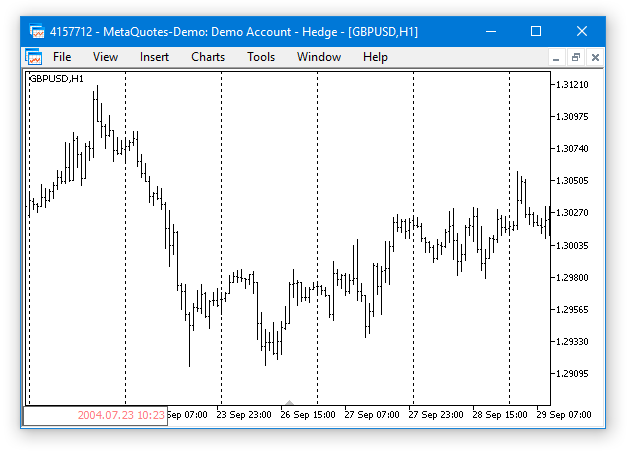Fig. 1. Command line of the chart at the left corner.

By the way, a new feature for managing the command line has been added in the latest update of the trading terminal (build 1455).

8. MQL5: New property CHART_QUICK_NAVIGATION allows enabling/disabling quick navigation bar in the chart. If you need to modify and access the property state, use the ChartSetInteger and ChartGetInteger functions.

The navigation bar is opened by pressing Enter or Space. It allows you to quickly move to the specified date on the chart, as well as to switch symbol and timeframe. If your MQL5 program processes Enter or Space key pressing, disable the CHART_QUICK_NAVIGATION property, to avoid interception of these events by the terminal. The quick navigation bar can still be opened by a double click.

…

Within the graphical interface, everything can be done more convenient and easier. The Easy And Fast library already contains the Calendar control (the CCalendar class). The main chart and subchart navigation can be implemented by simply choosing a date in the calendar. Let us simplify everything down to a single method with one argument. The value of this argument will be the date where chart needs to be shifted. This method will be named CStandardChart::SubChartNavigate(), the code listing below demonstrates the current version of this method.

The "Auto Scroll" and "Shift from the right border of the chart" modes of the main chart are disabled at the beginning of the method. Then, if the variable passed to the method is greater than the beginning of the current day, simply go to the last bar and leave the method. If the date is less, then it is necessary to calculate the number of bars for shifting to the left. First, the calculation is performed for the main chart:

• Get the total number of available bars for the current symbol and timeframe from the beginning of the current day until the specified date.
• Get the number of bars visible on the chart.
• Get the number of bars from the beginning of the current day + two bars as an additional indentation.
• Calculate the number of bars for shifting from the last bar.

After that, the main chart is shifted to the left, and everything is repeated for the subcharts.

```class CStandardChart : public CElement
{
public:
//--- Jump to the specified date
void              SubChartNavigate(const datetime date);
};
//+------------------------------------------------------------------+
//| Jump to the specified date                                       |
//+------------------------------------------------------------------+
void CStandardChart::SubChartNavigate(const datetime date)
{
//--- (1) The current chart date and (2) newly selected date in the calendar
datetime current_date  =::StringToTime(::TimeToString(::TimeCurrent(),TIME_DATE));
datetime selected_date =date;
//--- Disable auto scroll and shift from the right border
::ChartSetInteger(m_chart_id,CHART_AUTOSCROLL,false);
::ChartSetInteger(m_chart_id,CHART_SHIFT,false);
//--- If the date selected in the calendar is greater than the current date
if(selected_date>=current_date)
{
//--- Go to the current date on all charts
::ChartNavigate(m_chart_id,CHART_END);
ResetCharts();
return;
}
//--- Get the number of bars from the specified date
int  bars_total    =::Bars(::Symbol(),::Period(),selected_date,current_date);
int  visible_bars  =(int)::ChartGetInteger(m_chart_id,CHART_VISIBLE_BARS);
long seconds_today =::TimeCurrent()-current_date;
int  bars_today    =int(seconds_today/::PeriodSeconds())+2;
//--- Set the indent from the right border for all charts
m_prev_new_x_point=m_new_x_point=-((bars_total-visible_bars)+bars_today);
::ChartNavigate(m_chart_id,CHART_END,m_new_x_point);
//---
int sub_charts_total=SubChartsTotal();
for(int i=0; i<sub_charts_total; i++)
{
//--- Disable auto scroll and shift from the right border
::ChartSetInteger(m_sub_chart_id[i],CHART_AUTOSCROLL,false);
::ChartSetInteger(m_sub_chart_id[i],CHART_SHIFT,false);
//--- Get the number of bars from the specified date
bars_total   =::Bars(m_sub_chart[i].Symbol(),(ENUM_TIMEFRAMES)m_sub_chart[i].Period(),selected_date,current_date);
visible_bars =(int)::ChartGetInteger(m_sub_chart_id[i],CHART_VISIBLE_BARS);
bars_today   =int(seconds_today/::PeriodSeconds((ENUM_TIMEFRAMES)m_sub_chart[i].Period()))+2;
//--- Indent from the right border of the chart
m_prev_new_x_point=m_new_x_point=-((bars_total-visible_bars)+bars_today);
::ChartNavigate(m_sub_chart_id[i],CHART_END,m_new_x_point);
}
}
```

Development of the CStandardChart class for creating the Standard Chart Control is finished. Now let us write an application to see how it works.

### Application for testing the control

For testing purposes, you can use the Expert Advisor from the previous article. Remove all controls except the main menu, status bar and tabs. Make it so that each tab has a separate group of subcharts. Each group will contain a certain currency; therefore, each tab will have the corresponding description:

• The first tab - EUR (Euro).
• The second tab - GBP (Great Britain Pound).
• The third tab - AUD (Australian Dollar).
• The fourth tab - CAD (Canadian Dollar).
• The fifth tab - JPY (Japanese Yen).

The subcharts will be located strictly in the working area of the tabs and they will be automatically resized whenever the form is resized. The right border of the working area in the tabs will always have an indent of 173 pixels from the right edge of the form. This space will be filled with controls for setting such properties as:

• Displaying the time scale (Date time).
• Displaying the price scale (Price scale).
• Changing the chart timeframe (Timeframes).
• Navigating the chart data via the calendar.

As an example, it is sufficient to show the code for creating a single Standard Chart (CStandardChart) control. Remember that the horizontal scrolling of the charts is disabled by default, so in case it is needed, the CStandardChart::XScrollMode() method can be used. The CStandardChart::AddSubChart() method is used for adding charts to the group.

```//+------------------------------------------------------------------+
//| Class for creating the application                               |
//+------------------------------------------------------------------+
class CProgram : public CWndEvents
{
protected:
//--- Standard chart
CStandardChart    m_sub_chart1;
//---
protected:
//--- Standard chart
bool              CreateSubChart1(const int x_gap,const int y_gap);
};
//+------------------------------------------------------------------+
//| Create standard chart 1                                          |
//+------------------------------------------------------------------+
bool CProgram::CreateSubChart1(const int x_gap,const int y_gap)
{
//--- Store the window pointer
m_sub_chart1.WindowPointer(m_window);
//--- Attach to the first tab
m_tabs.AddToElementsArray(0,m_sub_chart1);
//--- Coordinates
int x=m_window.X()+x_gap;
int y=m_window.Y()+y_gap;
//--- Set properties before creation
m_sub_chart1.XSize(600);
m_sub_chart1.YSize(200);
m_sub_chart1.AutoXResizeMode(true);
m_sub_chart1.AutoYResizeMode(true);
m_sub_chart1.AutoXResizeRightOffset(175);
m_sub_chart1.AutoYResizeBottomOffset(25);
m_sub_chart1.XScrollMode(true);
//--- Add the charts
m_sub_chart1.AddSubChart("EURUSD",PERIOD_D1);
m_sub_chart1.AddSubChart("EURGBP",PERIOD_D1);
m_sub_chart1.AddSubChart("EURAUD",PERIOD_D1);
//--- Create control
if(!m_sub_chart1.CreateStandardChart(m_chart_id,m_subwin,x,y))
return(false);
//--- Add the object to the common array of object groups
CWndContainer::AddToElementsArray(0,m_sub_chart1);
return(true);
}
```

The screenshot below shows the final result. In this example, the subchart data can be scrolled horizontally, in a similar fashion to how it is implemented for the main chart. In addition, the navigation between subcharts works via the calendar, including fast forwarding the dates.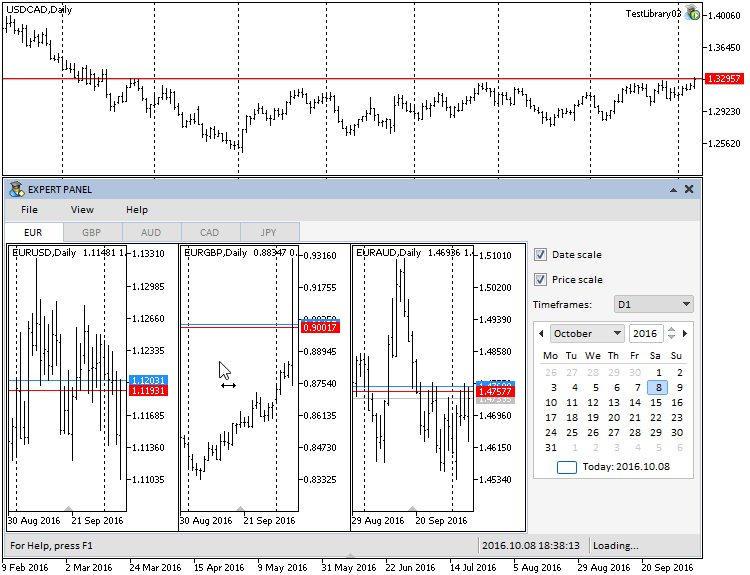Fig. 2. Test of the Standard Chart control.

The test application featured in the article can be downloaded using the below link for further studying.

### Optimization of timer and event handler of the library engine

Earlier, the Easy And Fast library had only been tested in the Windows 7 x64 operating system. After upgrading to Windows 10 x64, it was found that the CPU usage increases significantly. The library processes consumed up to 10% of the CPU resources even in the standby mode, when there was no interaction with the graphical interface. The screenshots below show the consumption of the CPU resources before and after attaching the test MQL application to the chart.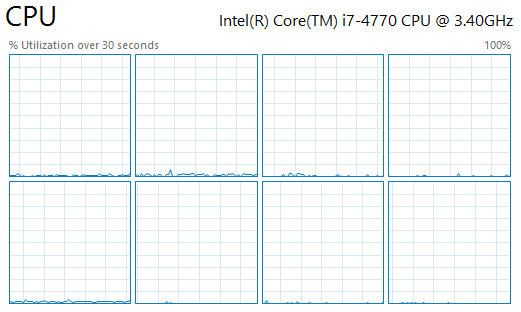Fig. 3. Consumption of the CPU resources before attaching the test MQL application to the chart.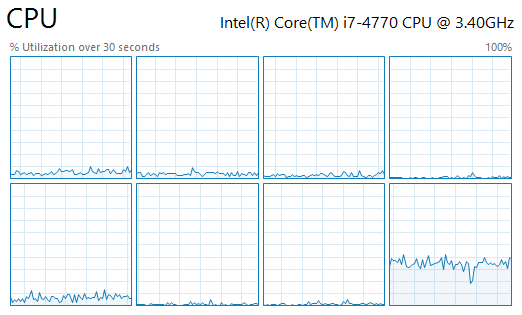Fig. 4. Consumption of the CPU resources after attaching the test MQL application to the chart.

It turned out that the problem was in the timer of the library engine, where the chart is updated every 16ms, as shown in the code below:

```//+------------------------------------------------------------------+
//| Timer                                                            |
//+------------------------------------------------------------------+
void CWndEvents::OnTimerEvent(void)
{
//--- Leave, if the array is empty
if(CWndContainer::WindowsTotal()<1)
return;
//--- Checking events of all elements by timer
CheckElementsEventsTimer();
//--- Redraw chart
m_chart.Redraw();
}
```

The CPU consumption increases even further, if we add the mouse cursor movements within the chart area and active interaction with the graphical interface of the MQL application. The task is to reduce operation of the library engine timer and eliminate redrawing the chart every time the mouse movement event is fired. How can this be done?

Delete the line responsible for redrawing the chart (highlighted in red) from the CWndEvents::ChartEventMouseMove() method:

```//+------------------------------------------------------------------+
//| CHARTEVENT MOUSE MOVE event                                      |
//+------------------------------------------------------------------+
void CWndEvents::ChartEventMouseMove(void)
{
//--- Leave, if this is not a cursor movement event
if(m_id!=CHARTEVENT_MOUSE_MOVE)
return;
//--- Moving the window
MovingWindow();
//--- Setting the chart state
SetChartState();
//--- Redraw chart
m_chart.Redraw();
}
```

As for the library engine timer, its current purpose comes down to changing the color of the controls when they are hovered by mouse and performing the fast forwarding different controls (lists, tables, calendar, etc.) Therefore, it has to be working constantly. To save resources, the timer will be activated when the mouse cursor starts moving, and as soon as the movement ends, its operation will be blocked after a brief pause.

In order to implement the idea, it is necessary to make some additions to the CMouse class. The additions include a counter for calls to the system timer and the CMouse::GapBetweenCalls() method, which returns the difference between the calls to the mouse movement events.

```class CMouse
{
private:
//--- Counter of calls
ulong             m_call_counter;
//---
public:
//--- Returns (1) the counter value stored during the last call and (2) the difference between the calls to the handler of the mouse movement events
ulong             CallCounter(void)     const { return(m_call_counter);                  }
ulong             GapBetweenCalls(void) const { return(::GetTickCount()-m_call_counter); }
};
//+------------------------------------------------------------------+
//| Constructor                                                      |
//+------------------------------------------------------------------+
CMouse::CMouse(void) : m_call_counter(::GetTickCount())
{
}
```

The logic is simple. As soon as the mouse cursor starts moving, the event handler of the CMouse class stores the current value of the system timer

```//+------------------------------------------------------------------+
//| Handle events of moving the mouse cursor                         |
//+------------------------------------------------------------------+
void CMouse::OnEvent(const int id,const long &lparam,const double &dparam,const string &sparam)
{
//--- Handling of the cursor movement event
if(id==CHARTEVENT_MOUSE_MOVE)
{
//--- Coordinates and the state of the left mouse button
m_x                 =(int)lparam;
m_y                 =(int)dparam;
m_left_button_state =(bool)int(sparam);
//--- Store the value of the calls counter
m_call_counter=::GetTickCount();
//--- Get the cursor location
if(!::ChartXYToTimePrice(0,m_x,m_y,m_subwin,m_time,m_level))
return;
//--- Get the relative Y coordinate
if(m_subwin>0)
m_y=m_y-m_chart.SubwindowY(m_subwin);
}
}
```

The timer of the library engine (the CWndEvents class) must contain a condition: if the mouse cursor has not been moved for over 500ms, the chart should not be redrawn. The left mouse button must be released at that time, to avoid the situation where the fast forward option for controls works only for 500ms.

```//+------------------------------------------------------------------+
//| Timer                                                            |
//+------------------------------------------------------------------+
void CWndEvents::OnTimerEvent(void)
{
//--- Leave, if the mouse cursor is at rest (difference between calls is greater than 500ms) and the left mouse button is released
if(m_mouse.GapBetweenCalls()>500 && !m_mouse.LeftButtonState())
return;
//--- Leave, if the array is empty
if(CWndContainer::WindowsTotal()<1)
return;
//--- Checking events of all elements by timer
CheckElementsEventsTimer();
//--- Redraw chart
m_chart.Redraw();
}
```

Problem solved. Disabling the redrawing whenever the mouse cursor moves had no effect on the quality of moving a form with controls, as the interval of 16ms in the timer is quite sufficient for redrawing. The problem has been solved in the simplest, but not the only possible way. The optimization of the library code will be discussed further in the upcoming articles of the series, as there are other methods and options that can help reduce the CPU consumption more efficiently.

### Optimization of the Tree View and File Navigator controls

It was found that the initialization took very long time for a tree view (CTreeView) containing a large number of elements. This also occurred in the file navigator (CFileNavigator), which utilizes this list type. To solve this problem, it is necessary to specify the reserve size for the array as the third parameter of the ::ArrayResize() function when adding elements to the arrays.

Quote from the ::ArrayResize() function reference:

With the frequent memory allocation, it is recommended to use a third parameter that sets a reserve to reduce the number of physical memory allocations. All the subsequent calls of ArrayResize do not lead to physical reallocation of memory, but only change the size of the first array dimension within the reserved memory. It should be remembered that the third parameter will be used only during physical memory allocation...

For comparison, below are the results of tests with different values of the reserve size for arrays in a tree view. The number of files for the test exceeds 15 000.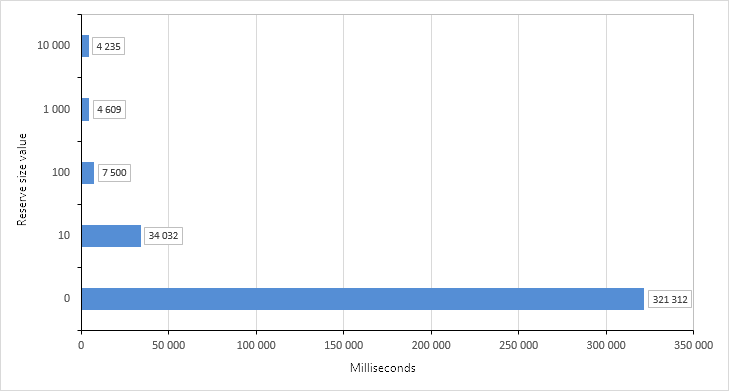Fig. 5. Results of tests for forming arrays with the reserve size values.

Set the reserve size for arrays of the tree view equal to 10 000. The appropriate changes have been made to the CTreeView and CFileNavigator classes.

### New icons for folders and files in the file navigator.

Added new icons for folders and files to the file navigator (the CFileNavigator class), which are analogous to the ones used in the file navigator of the Windows 10 operating system. Their sleek design is more suited for graphical interfaces of the library in development, but if necessary, custom versions can be used as well.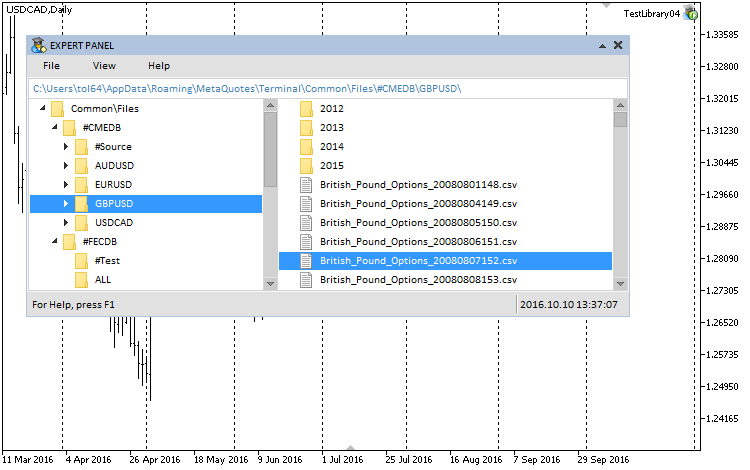Fig. 6. New icons for folders and files in the file navigator.

These images are available at the end of this article.

### Conclusion

The library for creating graphical interfaces at the current stage of development looks like in the schematic below.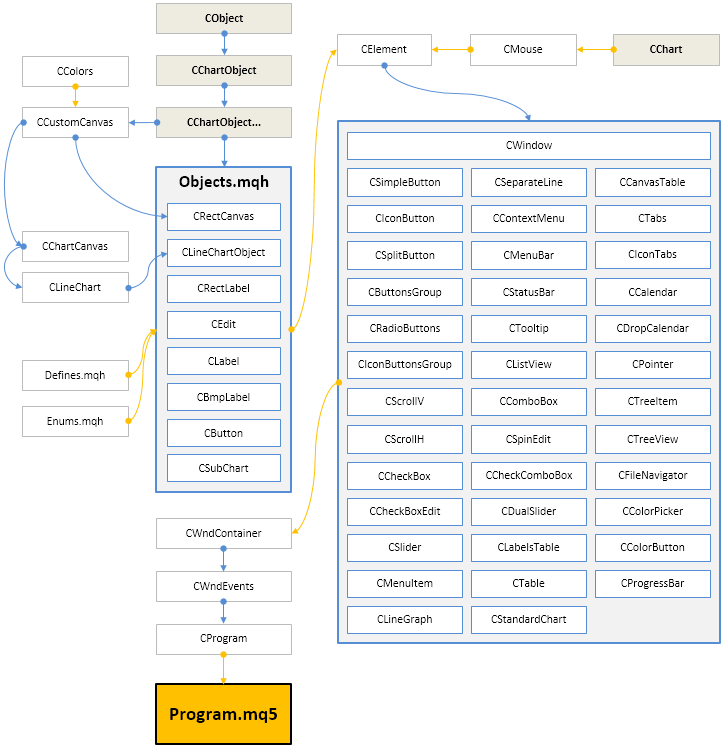Fig. 6. Structure of the library at the current stage of development.

The next articles on graphical interfaces will continue the development of the Easy And Fast library. The library will be expanded with additional controls, which may be necessary in development of MQL applications. The existing controls will be improved and supplemented with new features.

Below you can download the fourth version (build 4) of the Easy And Fast library. If you are interested, you can contribute to the faster development of this project by suggesting solutions to some tasks in the article comments or via private messages.

Translated from Russian by MetaQuotes Software Corp.
Original article: https://www.mql5.com/ru/articles/2763

Attached files |

#### Other articles by this author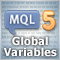MQL5 Programming Basics: Global Variables of the MetaTrader 5 Terminal
Global variables of the terminal provide an indispensable tool for developing sophisticated and reliable Expert Advisors. If you master the global variables, you will no more be able to imagine developing EAs on MQL5 without them.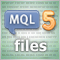MQL5 Programming Basics: Files
This practice-oriented article focuses on working with files in MQL5. It offers a number of simple tasks allowing you to grasp the basics and hone your skills.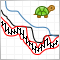The 'Turtle Soup' trading system and its 'Turtle Soup Plus One' modification
The article features formalized rules of two trading strategies 'Turtle Soup' and 'Turtle Soup Plus One' from Street Smarts: High Probability Short-Term Trading Strategies by Linda Bradford Raschke and Laurence A. Connors. The strategies described in the book are quite popular. But it is important to understand that the authors have developed them based on the 15...20 year old market behavior.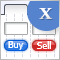Graphical Interfaces X: Updates for Easy And Fast Library (Build 3)
The next version of the Easy And Fast library (version 3) is presented in this article. Fixed certain flaws and added new features. More details further in the article.